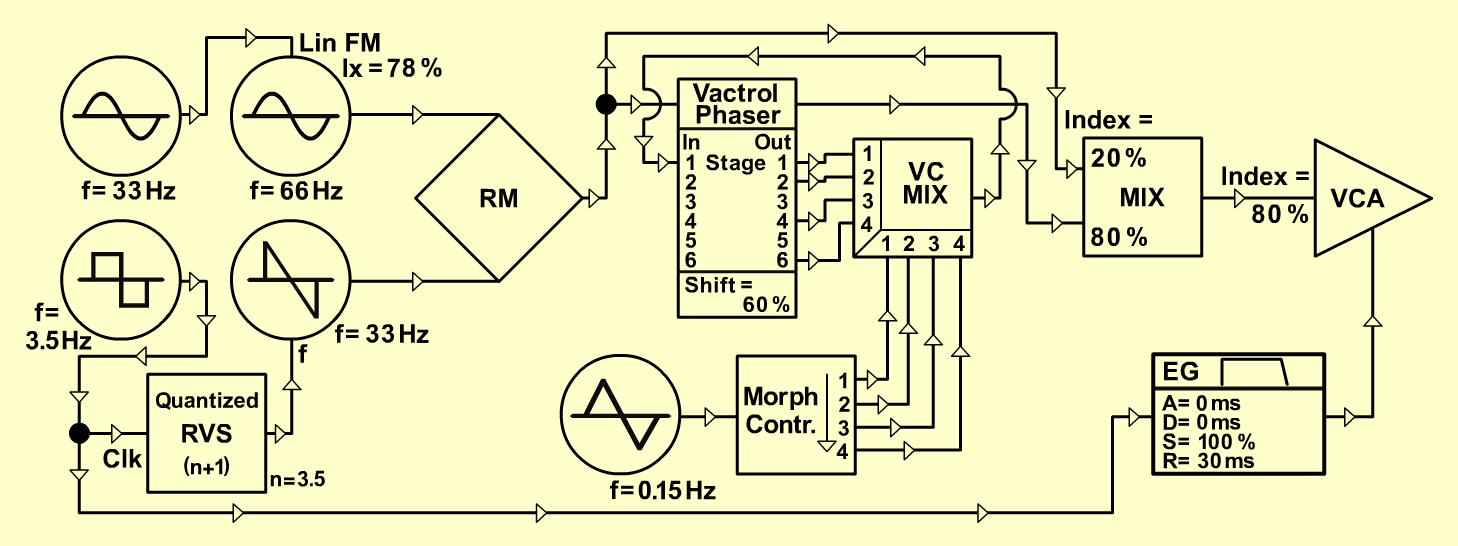Phase-FormatorTextureD o e p f e r A - 100 Connections: Settings: A-147/1 (Square) <=> A-180   A-180 <=> A-140 (Gate)   A-180 <=> A-149-1 (Quantized Clk In)   A-149-1 (n+1) <=> A-111/1 (CV1)   A-111/1 (Sine) <=> A-111/2 (Lin. FM)   A-111/1 (Saw) <=> A-114 (Y In)   A-111/2 (Sine) <=> A-114 (X In)   A-114 (Out) <=> A-101-3 (Audio In 1-6)   A-147/2 (Triangle) <=> A-101-3 (CV In 1-6)   A-101-3 (Mixed Out) <=> A-131 (Audio In)   A-140 (Output) <=> A-131 (CV 1)   A-149-1 (Stage Out 1) <=> A-135 (Audio In 1)   A-149-1 (Stage Out 2) <=> A-135 (Audio In 2)   A-149-1 (Stage Out 4) <=> A-135 (Audio In 3)   A-149-1 (Stage Out 6) <=> A-135 (Audio In 4)   A-135 (Audio Out) <=> A-101-3 (Polarizer 1 In)   A-145 (Triangle) <=> A-144 (CV In)   A-144 (Out 1) <=> A-135 (ext. CV 1)   A-144 (Out 2) <=> A-135 (ext. CV 2)   A-144 (Out 3) <=> A-135 (ext. CV 3)   A-144 (Out 4) <=> A-135 (ext. CV 4)   A-131 (Audio Out) <=> Amplifier A-147/1 (Freq = 6.1)   A-147/2 (Freq = 3.8)   A-140 (A = 0, D = 8, S = 10, R = 1, Range = M)   A-149-1 (Man N = 3.5)   A-111/1 (Oct = -1, Tune = 5, Fine = 5.5)   A-111/2 (Oct = 0, Tune = 5, Fine = 5.5, Lin. FM = 7.8)   A-145 (Frq = 1, Range = M)   A-135 (Audio In 1 - 4 = 10; Gain 1 - 4 = 0, ext. CV 1 - 4 = 10)   A-144 (CV = 10, Man. Morph = 5)   A-131 (Gain = 0, Audio In 1 = 8, Audio Out = 7)   A-101-3 (Lev. = 5, CV = 5, Shift = 6, Mix = 8, Pol. 1 = +5) Ingo Zobel, August 2004  www.selfoscillate.de  *Sound sample Phase-Formator